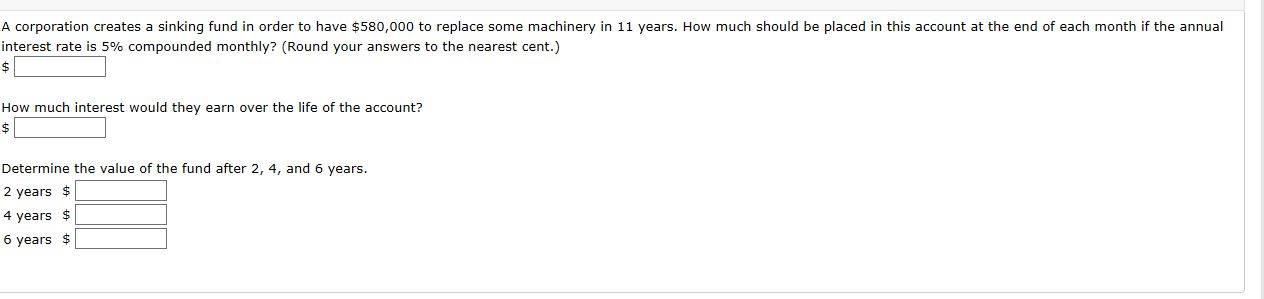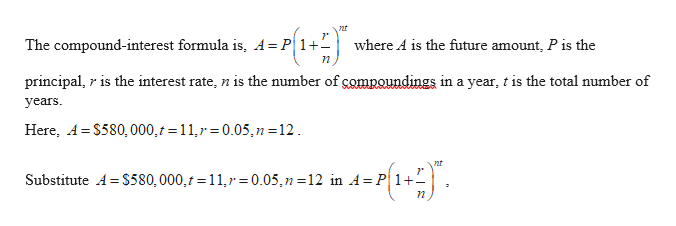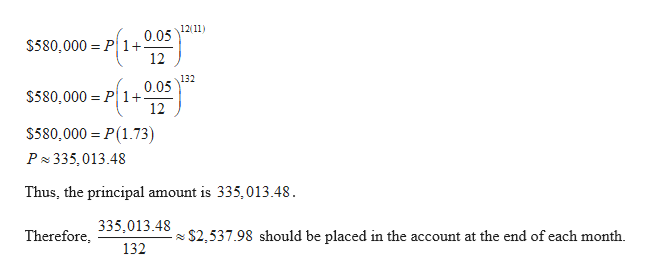# A corporation creates a sinking fund in order to have \$580,000 to replace some machinery in 11 years. How much should be placed in this account at the end of each month if the annualinterest rate is 5% compounded monthly? (Round your answers to the nearest cent.)\$How much interest would they earn over the life of the account?\$Determine the value of the fund after 2, 4, and 6 years.2 years \$4 years \$6 years \$

Question
22 viewshelp_outlineImage TranscriptioncloseA corporation creates a sinking fund in order to have \$580,000 to replace some machinery in 11 years. How much should be placed in this account at the end of each month if the annual interest rate is 5% compounded monthly? (Round your answers to the nearest cent.) \$ How much interest would they earn over the life of the account? \$ Determine the value of the fund after 2, 4, and 6 years. 2 years \$ 4 years \$ 6 years \$ fullscreen
check_circle

Step 1help_outlineImage Transcriptionclosent The compound-interest formula is, A= P1+1 where A is the future amount, P is the principal, r is the interest rate, n is the number of compoundings in a year, t is the total number of years Here, A S580, 000, t = 11, r = 0.05,n =12 Substitute A S580, 000, t = 11,r =0.05,n 12 in A= P1+1 n fullscreen
Step 2help_outlineImage Transcriptionclose12 11 0.05 \$580,000 P 1 12 132 0.05 \$580,000 P 1+ 12 \$580,000 P(1.73) P 335,013.48 Thus, the principal amount is 335,013.48 335,013.48 Therefore \$2,537.98 should be placed in the account at the end of each month 132 fullscreen
Step 3

### Want to see the full answer?

See Solution

#### Want to see this answer and more?

Solutions are written by subject experts who are available 24/7. Questions are typically answered within 1 hour.*

See Solution
*Response times may vary by subject and question.
Tagged in

### Other